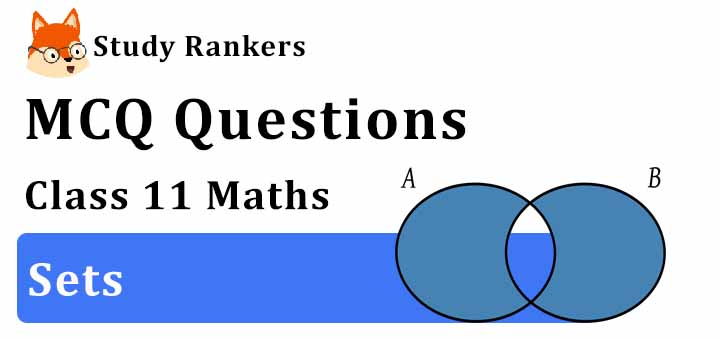# MCQ Questions for Class 11 Maths: Chapter 1 Sets with answers

Here you will get Class 11 Maths Chapter 1 Sets MCQ Questions with answers which is very helpful in knowing the topics in the chapter. MCQ Questions for Class 11 will guide in understanding the questions that can be asked in the exams and improve your marks.1. Write A = {1, 4, 9, 16, 25} in set builder form.
(a) A = {x : x is a prime number}
(b) A = {x : x is the cube of a natural number}
(c) A = {x : x is the square of a natural number}
(d) A = {x : x is an even natural number}
► (c) A = {x : x is the square of a natural number}

2. If A, B and C are any three sets, then A × (B ∪ C) is equal to
(a) (A × B) ∪ (A × C)
(b) (A ∪ B) × (A ∪ C)
(c) None of these
(d) (A × B) ∩ (A × C)
► (a) (A × B) ∪ (A × C)

3. If n (A) = 3 and n (B) = 6 and A ⊆ B, then the number of elements in A ∩ B is equal to
(a) 9
(b) 3
(c) 6
(d) 0
► (b) 3

4. IF R = {(2, 1),(4, 3),(4, 5)}, then range of the function is?
(a) Range R = {2, 4}
(b) Range R = {1, 3, 5}
(c) Range R = {2, 3, 4, 5}
(d) Range R {1, 1, 4, 5}
► (b) Range R = {1, 3, 5}

5. Let U = {1, 2, 3, 4, 5, 6, 7, 8, 9, 10}, A = {1, 2, 5}, B = {6, 7}. Then A ∩ B’ is :
(a) A
(b) B
(c) B’
(d) None of the above
► (a) A

6. The range of the function f(x) = 3x – 2‚ is
(a) (- ∞, ∞)
(b) R – {3}
(c) (- ∞, 0)
(d) (0, – ∞)
► (a) (- ∞, ∞)

7. How many rational and irrational numbers are possible between 0 and 1?
(a) 0
(b) Finite
(c) Infinite
(d) 1
► (c) Infinite

8. Which of the following two sets are equal?
(a) A = {1, 2} and B = {1}
(b) A = {1, 2} and B = {1, 2, 3}
(c) A = {1, 2, 3} and B = {2, 1, 3}
(d) A = {1, 2, 4} and B = {1, 2, 3}
► (c) A = {1, 2, 3} and B = {2, 1, 3}

9. Let A  and B be two sets then (A ∪ B)′ ∪ (A′ ∩ B) is equal to
(a) A'
(b) A
(c) B'
(d) None of these
► (a) A'

10. If (x – 1, y + 1) = (5, 6), then the value of x and y is given by
(a) x = 5, y = 5
(b) x = 6, y = 5
(c) x = 5, y = 6
(d) x = 0, y = 0
► (b) x = 6, y = 5

11. The cardinality of the power set of {0, 1, 2 . . ., 10} is ______.
(a) 1024
(b) 1023
(c) 2048
(d) 2043
► (c) 2048

12. If the sets A and B are defined as A = {(x, y) : y = ex, x ∈ R}; B = {(x, y) : y = x,x ∈ R}, then
(a) B ⊆ A
(b) A ⊆ B
(c) A ∪ B = A
(d) A ∩ B = φ
► (d) A ∩ B = φ

13. The range of the function f(x) = 3x – 2‚ is
(a) (- ∞, ∞)
(b) R – {3}
(c) (- ∞, 0)
(d) (0, – ∞)
► (a) (- ∞, ∞)

14. If A = {1, 2, 3, 4}, B = {4, 5, 6, 7}, then A - B =
(a) {5, 6, 7}
(b) {1, 2, 3, 4}
(c) {4}
(d) {1, 2, 3}
► (d) {1, 2, 3}

15. The set of intelligent students in a class is
(a) A finite set
(b) A null set
(c) Not a well defined collection
(d) A singleton set
► (c) Not a well defined collection

16. If A = {3, 6, 9, 12} and B = {6, 8, 9} then intersection of A and B is
(a) {3, 6}
(b) {3, 12}
(c) {6, 9}
(d) {9, 12}
► (c) {6, 9}

17. If set A has 4 elements and B = {5, 6}, then the number of elements in A x B are
(a) 9
(b) 8
(c) 7
(d) 10
► (b) 8

18. If A = {1, 2, 4}, B = {2, 4, 5}, C = {2, 5}, then (A - B) × (B - C) is
(a) { (1, 2), (1, 5), (2, 5) }
(b) { (1, 2), (1, 5), (2, 5), (2,4) }
(c) (1,4)
(d) { (1, 4) }
► (d) { (1, 4) }

19. Empty set is a?
(a) Finite Set
(b) Invalid Set
(c) None of the above
(d) Infinite Set
► (a) Finite Set

20. Which of the following two sets are equal?
(a) A = {1, 2} and B = {1}
(b) A = {1, 2} and B = {1, 2, 3}
(c) A = {1, 2, 3} and B = {2, 1, 3}
(d) A = {1, 2, 4} and B = {1, 2, 3}
► (c) A = {1, 2, 3} and B = {2, 1, 3}